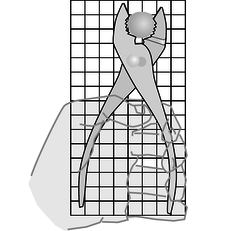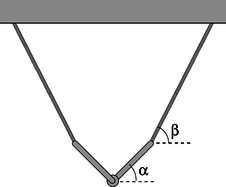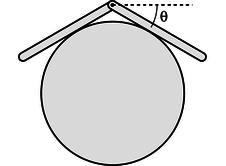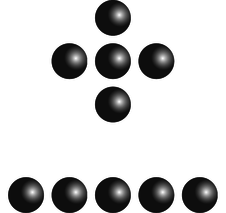# 5.E: Conservation of Angular Momentum (Exercises)

$$\newcommand{\vecs}{\overset { \rightharpoonup} {\mathbf{#1}} }$$ $$\newcommand{\vecd}{\overset{-\!-\!\rightharpoonup}{\vphantom{a}\smash {#1}}}$$$$\newcommand{\id}{\mathrm{id}}$$ $$\newcommand{\Span}{\mathrm{span}}$$ $$\newcommand{\kernel}{\mathrm{null}\,}$$ $$\newcommand{\range}{\mathrm{range}\,}$$ $$\newcommand{\RealPart}{\mathrm{Re}}$$ $$\newcommand{\ImaginaryPart}{\mathrm{Im}}$$ $$\newcommand{\Argument}{\mathrm{Arg}}$$ $$\newcommand{\norm}{\| #1 \|}$$ $$\newcommand{\inner}{\langle #1, #2 \rangle}$$ $$\newcommand{\Span}{\mathrm{span}}$$ $$\newcommand{\id}{\mathrm{id}}$$ $$\newcommand{\Span}{\mathrm{span}}$$ $$\newcommand{\kernel}{\mathrm{null}\,}$$ $$\newcommand{\range}{\mathrm{range}\,}$$ $$\newcommand{\RealPart}{\mathrm{Re}}$$ $$\newcommand{\ImaginaryPart}{\mathrm{Im}}$$ $$\newcommand{\Argument}{\mathrm{Arg}}$$ $$\newcommand{\norm}{\| #1 \|}$$ $$\newcommand{\inner}{\langle #1, #2 \rangle}$$ $$\newcommand{\Span}{\mathrm{span}}$$$$\newcommand{\AA}{\unicode[.8,0]{x212B}}$$a / Problem 1.

1. The figure shows scale drawing of a pair of pliers being used to crack a nut, with an appropriately reduced centimeter grid. Warning: do not attempt this at home; it is bad manners. If the force required to crack the nut is 300 N, estimate the force required of the person's hand. (solution in the pdf version of the book)

2. You are trying to loosen a stuck bolt on your RV using a big wrench that is 50 cm long. If you hang from the wrench, and your mass is 55 kg, what is the maximum torque you can exert on the bolt? (answer check available at lightandmatter.com)

3. A physical therapist wants her patient to rehabilitate his injured elbow by laying his arm flat on a table, and then lifting a 2.1 kg mass by bending his elbow. In this situation, the weight is 33 cm from his elbow. He calls her back, complaining that it hurts him to grasp the weight. He asks if he can strap a bigger weight onto his arm, only 17 cm from his elbow. How much mass should she tell him to use so that he will be exerting the same torque? (He is raising his forearm itself, as well as the weight.) (answer check available at lightandmatter.com)

4. An object thrown straight up in the air is momentarily at rest when it reaches the top of its motion. Does that mean that it is in equilibrium at that point? Explain.

5. An object is observed to have constant angular momentum. Can you conclude that no torques are acting on it? Explain. [Based on a problem by Serway and Faughn.]

{C}{C}b / Problem 6.

6. A person of mass $$m$$ stands on the ball of one foot. Find the tension in the calf muscle and the force exerted by the shinbones on the bones of the foot, in terms of $$m$$, $$g$$, $$a$$, and $$b$$. For simplicity, assume that all the forces are at 90-degree angles to the foot, i.e., neglect the angle between the foot and the floor.(answer check available at lightandmatter.com)

7. Two pointlike particles have the same momentum vector. Can you conclude that their angular momenta are the same? Explain. [Based on a problem by Serway and Faughn.]

{C}{C}c / Problem 8.

8. The box shown in the figure is being accelerated by pulling on it with the rope.
(a) Assume the floor is frictionless. What is the maximum force that can be applied without causing the box to tip over?\hwhint{hwhint:tipbox}(answer check available at lightandmatter.com)
(b) Repeat part a, but now let the coefficient of friction be $$\mu$$.(answer check available at lightandmatter.com)
(c) What happens to your answer to part b when the box is sufficiently tall? How do you interpret this?

9. A uniform ladder of mass $$m$$ and length $$\ell$$ leans against a smooth wall, making an angle $$\theta$$ with respect to the ground. The dirt exerts a normal force and a frictional force on the ladder, producing a force vector with magnitude $$F_1$$ at an angle $$\phi$$ with respect to the ground. Since the wall is smooth, it exerts only a normal force on the ladder; let its magnitude be $$F_2$$.
(a) Explain why $$\phi$$ must be greater than $$\theta$$. No math is needed.
(b) Choose any numerical values you like for $$m$$ and $$\ell$$, and show that the ladder can be in equilibrium (zero torque and zero total force vector) for $$\theta$$=45.00° and $$\phi$$=63.43°.

{C}{C}d / Problems 9 and 10.

10. Continuing problem 9, find an equation for $$\phi$$ in terms of $$\theta$$, and show that $$m$$ and $$L$$ do not enter into the equation. Do not assume any numerical values for any of the variables. You will need the trig identity $$\sin(a-b) = \sin a \cos b - \sin b \cos a$$. (As a numerical check on your result, you may wish to check that the angles given in problem 9b satisfy your equation.)(answer check available at lightandmatter.com)

{C}{C}e / Problem 11.

11. (a) Find the minimum horizontal force which, applied at the axle, will pull a wheel over a step. Invent algebra symbols for whatever quantities you find to be relevant, and give your answer in symbolic form.
(b) Under what circumstances does your result become infinite? Give a physical interpretation. What happens to your answer when the height of the curb is zero? Does this make sense? \hwhint{hwhint:wheeloverstep}

12. A ball is connected by a string to a vertical post. The ball is set in horizontal motion so that it starts winding the string around the post. Assume that the motion is confined to a horizontal plane, i.e., ignore gravity. Michelle and Astrid are trying to predict the final velocity of the ball when it reaches the post. Michelle says that according to conservation of angular momentum, the ball has to speed up as it approaches the post. Astrid says that according to conservation of energy, the ball has to keep a constant speed. Who is right? [Hint: How is this different from the case where you whirl a rock in a circle on a string and gradually reel in the string?]

13. In the 1950's, serious articles began appearing in magazines like Life predicting that world domination would be achieved by the nation that could put nuclear bombs in orbiting space stations, from which they could be dropped at will. In fact it can be quite difficult to get an orbiting object to come down. Let the object have energy $$E=K+U$$ and angular momentum $$L$$. Assume that the energy is negative, i.e., the object is moving at less than escape velocity. Show that it can never reach a radius less than

$\begin{equation*} r_{min} = \frac{GMm}{2E}\left(-1+\sqrt{1+\frac{2EL^2}{G^2M^2m^3}}\right) . \end{equation*}$

[Note that both factors are negative, giving a positive result.]f / Problem 14.

14. (a) The bar of mass $$m$$ is attached at the wall with a hinge, and is supported on the right by a massless cable. Find the tension, $$T$$, in the cable in terms of the angle $$\theta$$.(answer check available at lightandmatter.com)
(b) Interpreting your answer to part a, what would be the best angle to use if we wanted to minimize the strain on the cable?
(c) Again interpreting your answer to part a, for what angles does the result misbehave mathematically? Interpet this physically.g / Problem 15.

15. (a) The two identical rods are attached to one another with a hinge, and are supported by the two massless cables. Find the angle $$\alpha$$ in terms of the angle $$\beta$$, and show that the result is a purely geometric one, independent of the other variables involved.(answer check available at lightandmatter.com)
(b) Using your answer to part a, sketch the configurations for $$\beta\rightarrow 0$$, $$\beta= 45°$$, and $$\beta= 90°$$. Do your results make sense intuitively?h / Problem 16.

16. Two bars of length $$\ell$$ are connected with a hinge and placed on a frictionless cylinder of radius $$r$$. (a) Show that the angle $$\theta$$ shown in the figure is related to the unitless ratio $$r/\ell$$ by the equation

$\begin{equation*} \frac{r}{\ell} = \frac{\cos^2\theta}{2\:\tan\theta} . \end{equation*}$

(b) Discuss the physical behavior of this equation for very large and very small values of $$r/\ell$$.i / Problem 17.

17. You wish to determine the mass of a ship in a bottle without taking it out. Show that this can be done with the setup shown in the figure, with a scale supporting the bottle at one end, provided that it is possible to take readings with the ship slid to several different locations. Note that you can't determine the position of the ship's center of mass just by looking at it, and likewise for the bottle. In particular, you can't just say, “position the ship right on top of the fulcrum” or “position it right on top of the balance.”

18. Suppose that we lived in a universe in which Newton's law of gravity gave an interaction energy proportional to $$r^{-6}$$, rather than $$r^{-1}$$. Which, if any, of Kepler's laws would still be true? Which would be completely false? Which would be different, but in a way that could be calculated with straightforward algebra?

19. Use analogies to find the equivalents of the following equations for rotation in a plane:

\begin{align*} KE &= p^2/2m \\ \Delta x &= v_\text{o}\Delta t + (1/2)a\Delta t^2\\ W &= F \Delta x \end{align*}

Example: $$v = \Delta x/\Delta t \rightarrow \omega = \Delta\theta/\Delta t$$

20. For a one-dimensional harmonic oscillator, the solution to the energy conservation equation,

$\begin{equation*} U+K = \frac{1}{2}kx^2+\frac{1}{2}mv^2 = \text{constant} , \end{equation*}$

is an oscillation with frequency $$\omega=\sqrt{k/m}$$.

Now consider an analogous system consisting of a bar magnet hung from a thread, which acts like a magnetic compass. A normal compass is full of water, so its oscillations are strongly damped, but the magnet-on-a-thread compass has very little friction, and will oscillate repeatedly around its equilibrium direction. The magnetic energy of the bar magnet is

$\begin{equation*} U = -Bm\cos\theta , \end{equation*}$

where $$B$$ is a constant that measures the strength of the earth's magnetic field, $$m$$ is a constant that parametrizes the strength of the magnet, and $$\theta$$ is the angle, measured in radians, between the bar magnet and magnetic north. The equilibrium occurs at $$\theta=0$$, which is the minimum of $$U$$.

(a) Problem 19 on p. 291 gave some examples of how to construct analogies between rotational and linear motion. Using the same technique, translate the equation defining the linear quantity $$k$$ to one that defines an analogous angular one $$\kappa$$ (Greek letter kappa). Applying this to the present example, find an expression for $$\kappa$$. (Assume the thread is so thin that its stiffness does not have any significant effect compared to earth's magnetic field.)
(b) Find the frequency of the compass's vibrations.

21. (a) Find the angular velocities of the earth's rotation and of the earth's motion around the sun.(answer check available at lightandmatter.com)
(b) Which motion involves the greater acceleration?j / Problem 22.

22. The sun turns on its axis once every 26.0 days. Its mass is $$2.0\times10^{30}$$ kg and its radius is $$7.0\times10^8$$ m. Assume it is a rigid sphere of uniform density.
(a) What is the sun's angular momentum? (answer check available at lightandmatter.com)
In a few billion years, astrophysicists predict that the sun will use up all its sources of nuclear energy, and will collapse into a ball of exotic, dense matter known as a white dwarf. Assume that its radius becomes $$5.8\times10^6$$ m (similar to the size of the Earth.) Assume it does not lose any mass between now and then. (Don't be fooled by the photo, which makes it look like nearly all of the star was thrown off by the explosion. The visually prominent gas cloud is actually thinner than the best laboratory vacuum ever produced on earth. Certainly a little bit of mass is actually lost, but it is not at all unreasonable to make an approximation of zero loss of mass as we are doing.)
(b) What will its angular momentum be?
(c) How long will it take to turn once on its axis? (answer check available at lightandmatter.com)k / Problem 23

23. Give a numerical comparison of the two molecules' moments of inertia for rotation in the plane of the page about their centers of mass.

24. A yo-yo of total mass $$m$$ consists of two solid cylinders of radius $$R$$, connected by a small spindle of negligible mass and radius $$r$$. The top of the string is held motionless while the string unrolls from the spindle. Show that the acceleration of the yo-yo is $$g/(1+R^2/2r^2)$$.

25. Show that a sphere of radius $$R$$ that is rolling without slipping has angular momentum and momentum in the ratio $$L/p=(2/5)R$$.

26. Suppose a bowling ball is initially thrown so that it has no angular momentum at all, i.e., it is initially just sliding down the lane. Eventually kinetic friction will bring its angular velocity up to the point where it is rolling without slipping. Show that the final velocity of the ball equals 5/7 of its initial velocity. You'll need the result of problem 25.

27. Find the angular momentum of a particle whose position is $$\mathbf{r}=3\hat{\mathbf{x}}-\hat{\mathbf{y}}+\hat{\mathbf{z}}$$ (in meters) and whose momentum is $$\mathbf{p}=-2\hat{\mathbf{x}}+\hat{\mathbf{y}}+\hat{\mathbf{z}}$$ (in $$\text{kg}\!\cdot\!\text{m}/\text{s}$$). (answer check available at lightandmatter.com)

28. Find a vector that is perpendicular to both of the following two vectors:

$\begin{gather*} \hat{\mathbf{x}}+2\hat{\mathbf{y}}+3\hat{\mathbf{z}} \\ 4\hat{\mathbf{x}}+5\hat{\mathbf{y}}+6\hat{\mathbf{z}} \end{gather*}$

29. Prove property (3) of the vector cross product from the theorem on page 912.

30. Prove the anticommutative property of the vector cross product, $$\mathbf{A}\times\mathbf{B} = -\mathbf{B}\times\mathbf{A}$$, using the expressions for the components of the cross product.

31. Find three vectors with which you can demonstrate that the vector cross product need not be associative, i.e., that $$\mathbf{A}\times(\mathbf{B}\times\mathbf{C})$$ need not be the same as $$(\mathbf{A}\times\mathbf{B})\times\mathbf{C}$$.

32. Which of the following expressions make sense, and which are nonsense? For those that make sense, indicate whether the result is a vector or a scalar.
(a) $$(\mathbf{A}\times\mathbf{B})\times\mathbf{C}$$
(b) $$(\mathbf{A}\times\mathbf{B})\cdot\mathbf{C}$$
(c) $$(\mathbf{A}\cdot\mathbf{B})\times\mathbf{C}$$l / Problem 33

33. (a) As suggested in the figure, find the area of the infinitesimal region expressed in polar coordinates as lying between $$r$$ and $$r+dr$$ and between $$\theta$$ and $$\theta+d\theta$$.(answer check available at lightandmatter.com)
(b) Generalize this to find the infinitesimal element of volume in cylindrical coordinates $$(r,\theta,z)$$, where the Cartesian $$z$$ axis is perpendicular to the directions measured by $$r$$ and $$\theta$$.(answer check available at lightandmatter.com)
(c) Find the moment of inertia for rotation about its axis of a cone whose mass is $$M$$, whose height is $$h$$, and whose base has a radius $$b$$.(answer check available at lightandmatter.com)

34. Find the moment of inertia of a solid rectangular box of mass $$M$$ and uniform density, whose sides are of length $$a$$, $$b$$, and $$c$$, for rotation about an axis through its center parallel to the edges of length $$a$$.(answer check available at lightandmatter.com)

35. The nucleus $$^{168}\text{Er}$$ (erbium-168) contains 68 protons (which is what makes it a nucleus of the element erbium) and 100 neutrons. It has an ellipsoidal shape like an American football, with one long axis and two short axes that are of equal diameter. Because this is a subatomic system, consisting of only 168 particles, its behavior shows some clear quantum-mechanical properties. It can only have certain energy levels, and it makes quantum leaps between these levels. Also, its angular momentum can only have certain values, which are all multiples of $$2.109\times10^{-34}\ \text{kg}\cdot\text{m}^2/\text{s}$$. The table shows some of the observed angular momenta and energies of $$^{168}\text{Er}$$, in SI units ($$\text{kg}\cdot\text{m}^2/\text{s}$$ and joules).

 L×1034 E×1014 0 0 2.109 1.2786 4.218 4.2311 6.327 8.7919 8.437 14.8731 10.546 22.3798 12.655 31.135 14.764 41.206 16.873 52.223

(a) These data can be described to a good approximation as a rigid end-over-end rotation. Estimate a single best-fit value for the moment of inertia from the data, and check how well the data agree with the assumption of rigid-body rotation.\hwhint{hwhint:erbium}(answer check available at lightandmatter.com)
(b) Check whether this moment of inertia is on the right order of magnitude. The moment of inertia depends on both the size and the shape of the nucleus. For the sake of this rough check, ignore the fact that the nucleus is not quite spherical. To estimate its size, use the fact that a neutron or proton has a volume of about $$1\ \text{fm}^3$$ (one cubic femtometer, where $$1\ \text{fm}=10^{-15}\ \text{m}$$), and assume they are closely packed in the nucleus.

36. (a) Prove the identity $$\mathbf{a}\times(\mathbf{b}\times\mathbf{c})=\mathbf{b}(\mathbf{a}\cdot\mathbf{c})-\mathbf{c}(\mathbf{a}\cdot\mathbf{b})$$ by expanding the product in terms of its components. Note that because the $$x$$, $$y$$, and $$z$$ components are treated symmetrically in the definitions of the vector cross product, it is only necessary to carry out the proof for the $$x$$ component of the result.
(b) Applying this to the angular momentum of a rigidly rotating body, $$L=\int \mathbf{r}\times(\boldsymbol{\omega}\times\mathbf{r})dm$$, show that the diagonal elements of the moment of inertia tensor can be expressed as, e.g., $$I_{xx}=\int (y^2+z^2)dm$$.
(c) Find the diagonal elements of the moment of inertia matrix of an ellipsoid with axes of lengths $$a$$, $$b$$, and $$c$$, in the principal-axis frame, and with the axis at the center.(answer check available at lightandmatter.com)

37. In example 22 on page 276, prove that if the rod is sufficiently thin, it can be toppled without scraping on the floor. (solution in the pdf version of the book)

38. Suppose an object has mass $$m$$, and moment of inertia $$I_\text{o}$$ for rotation about some axis A passing through its center of mass. Prove that for an axis B, parallel to A and lying at a distance $$h$$ from it, the object's moment of inertia is given by $$I_\text{o}+mh^2$$. This is known as the parallel axis theorem.

39. Let two sides of a triangle be given by the vectors $$\mathbf{A}$$ and $$\mathbf{B}$$, with their tails at the origin, and let mass $$m$$ be uniformly distributed on the interior of the triangle. (a) Show that the distance of the triangle's center of mass from the intersection of sides $$\mathbf{A}$$ and $$\mathbf{B}$$ is given by $$\frac{1}{3}|\mathbf{A}+\mathbf{B}|$$.
(b) Consider the quadrilateral with mass $$2m$$, and vertices at the origin, $$\mathbf{A}$$, $$\mathbf{B}$$, and $$\mathbf{A}+\mathbf{B}$$. Show that its moment of inertia, for rotation about an axis perpendicular to it and passing through its center of mass, is $$\frac{m}{6}(A^2+B^2)$$.
(c) Show that the moment of inertia for rotation about an axis perpendicular to the plane of the original triangle, and passing through its center of mass, is $$\frac{m}{18}(A^2+B^2-\mathbf{A}\cdot\mathbf{B})$$. Hint: Combine the results of parts a and b with the result of problem 38.

40. When we talk about rigid-body rotation, the concept of a perfectly rigid body can only be an idealization. In reality, any object will compress, expand, or deform to some extent when subjected to the strain of rotation. However, if we let it settle down for a while, perhaps it will reach a new equilibrium. As an example, suppose we fill a centrifuge tube with some compressible substance like shaving cream or Wonder Bread. We can model the contents of the tube as a one-dimensional line of mass, extending from $$r=0$$ to $$r=\ell$$. Once the rotation starts, we expect that the contents will be most compressed near the “floor” of the tube at $$r=\ell$$; this is both because the inward force required for circular motion increases with $$r$$ for a fixed $$\omega$$, and because the part at the floor has the greatest amount of material pressing “down” (actually outward) on it. The linear density $$dm/dr$$, in units of kg/m, should therefore increase as a function of $$r$$. Suppose that we have $$dm/dr=\mu e^{r/\ell}$$, where $$\mu$$ is a constant. Find the moment of inertia.(answer check available at lightandmatter.com)

41. When we release an object such as a bicycle wheel or a coin on an inclined plane, we can observe a variety of different behaviors. Characterize these behaviors empirically and try to list the physical parameters that determine which behavior occurs. Try to form a conjecture about the behavior using simple closed-form expressions. Test your conjecture experimentally.